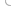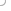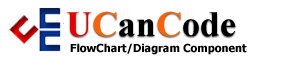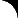YOU CAN CODE!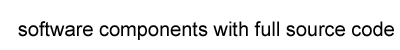With The Case Of UCanCode.net  Release The Power OF  Visual C++ ! Home |  Products | Purchase |  Support | DownloadsView in English
View in Japanese
View in
참고
View in Français
View in Italiano
View in 中文(繁體)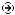Download EvaluationPricing & Purchase?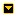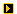E-XD++Visual C++/ MFC ProductsOverviewElectronic Form SolutionVisualization & HMI SolutionPower system HMI SolutionBar code labeling SolutionWorkflow SolutionCoal industry HMI SolutionInstrumentation Gauge SolutionReport Printing SolutionGraphical modeling SolutionGIS mapping solutionVisio graphics solutionIndustrial monitoring SolutionFlowchart and diagramming SolutionOrganization Diagram SolutionGraphic editor Source CodeUML drawing editor Source CodeMap Diagramming SolutionArchitectural Graphic Drawing SolutionRequest EvaluationActiveX COM ProductsOverviewTechnical Support# Database Programming with C/C++, with mySQL

Creating a database application in C/C++ is a daunting tucancode.net, especially for a novice programmer. Although the actually code is quite simple, it is the configuration issues such as importing right library, drivers to use, how to access them, and so forth, that make it an uphill battle. Modern high-level languages are pretty straightforward in these matters. They are quite simple and convenient with an all-in-one-place library with very few configuration troubles. One of the interesting aspects of using a high-level language is that you can almost start coding with even a little understanding of the underlying principles. C/C++, on the other hand, is a different breed. It is not easy to tread even a step superficially. This makes it more intriguing, challenging, that which tests your skills with every code you write. But, once you overcome the preliminary hurdles, there is nothing like C/C++. And the database programming? It's quite fun, actually. Let's get a first ride with the database code in C/C++ in this article.

## An Overview

There are many practical ways to access a database in C/C++. Except for ODBC; its APIs are not standard. Most database vendors provide a native client library to access a database. Client libraries are vendor specific; that means that the API provided by one is different from another although the underlying principles are the same. MySQL, for example, has its own client library and the API supplied by is are quite different from the API supplied by the client library of PostgreSQL. If you stick to one vendor-specific database, say MySQL, the driver options for database programming with C/C++ are:

• MySQL Client library: It is a native C API library distributed with MySQL and implemented in thelibmysqlclient library. If you have MySQL Server installed, most probably the client API library is already installed. (Otherwise, it may be downloaded with the command, something like sudo apt-get install libmysqlclient<version> libmysqlclient<version>-dev in Ubuntu/Mint.)
• MySQL C/C++ Connector: It is an innovation in its prime to make database connectivity simple and convenient. The API is based partially on the JDBC4.0 API standard and perhaps will be the standard way to access a database as it matures. There is a separate connector for C and as well as for C++. While using the C++ connector, no C functions calls are required.
• ODBC (Open Database Connectivity): Developed by Microsoft in the 90's, it provides a vendor-neutral API to a access database system. Most database vendors provide at least an ODBC driver apart from its native support. ODBC is basically a driver model that contains logic to convert a standard set of commands into calls understood by the underlying system. It stands between the application and the database system to reciprocate the exchange of calls/responses among them. In recent years, due to the advent of thin client computing, the requirement of virtualization offered by ODBC has been reduced. Most Web development platforms work in layers with HTML as the intermediary between the user and the application. And, in most cases, the underlying database layers have a direct link with the target database. This made native library more preferable over ODBC. Due to these reasons, the development of ODBC has slacked down in the recent years.

## C/C++ and MySQL

Let's try out a database application using a very basic, low-level MySQL client C API library. The database access routine more or less involves the following steps:

### 1. Initialize Connection Handle Structure

` `
1. MYSQL *mysql_init(MYSQL *);
` `

### 2. Make the Connection

` `
1. MYSQL mysql_real_connect(
2. MYSQL connection,
3. const char *host,
6. const char *database_name,
7. unsigned int port,
8. const char *unix_socket_name,
9. unsigned int flags
10. );
` `

### 3. Execute SQL Statements

` `
1. int mysql_query(MYSQL *connection, const char *query);
` `

### 4. Functions to Use for Data Retrieval

` `
1. MYSQL_RES *mysql_use_result(MYSQL *connection);
2.
3. MYSQL_ROW mysql_fetch_row(MYSQL_RES *result);
` `

### 5. Error Handling

` `
1. unsigned int mysql_errno(MYSQL *connection);
2.
3. char *mysql_error(MYSQL *connection);
` `

### 6. Close the Connection

` `
1. void mysql_close(MYSQL *connection);
` `

There are many other functions, but these are the functions we shall use when creating the application down the line. Consult the MySQl C API manuals for more details on these and other APIs. To sum up, you'll basically need at least the following software.

 Application Name Source Details Database MySQL 5 MySQL Database Server will be our back-end database Database API MySQL client library Provides native driver and library:libmysqlclient as an interface between the application and the database. Make sure that the client API library is installed. Once installed, the header files and the library are generally found in /usr/include/mysql and/usr/lib/mysql, respectively; otherwise, make sure of the correct path in your system. Compiler g++ GNU C++ compiler IDE CodeLite 9.1.8 It is not absolute necessary to use an IDE, but it is convenient to use one. There are many IDEs available in Linux for C/C++ programming. CodeLite seemed (to me) modern,  simple, and intuitive. Also, it creates the make file automatically. You may choose any other, even simple gedit,vi, or any other simple text editor such as nano is also fine. In such a case. consult the appropriate manual for the configuration and settings and how to create the make file if you resort to do everything manually.

## An Example: Transaction Processing System

This is a very simple and rudimentary implementation of a transaction processing system. The code could have been written in a C style (without classes) because the MySQL API functions calls are in C format. But, to get a feel of object-oriented database programming with C++, classes are used. In many cases, we had to force its parameters to go with the essence of C++ by casting and converting occasionally. The application processing can imagined as shown in Figure 1.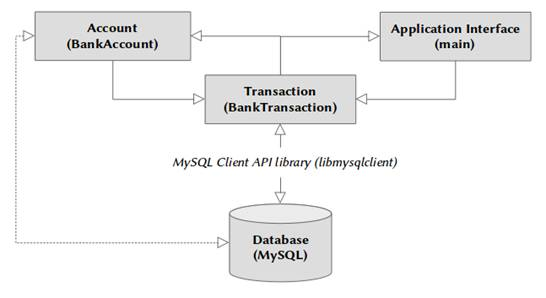Figure 1: Transaction Processing System

## Configuration and Settings the in IDE: CodeLite

Make sure the following configurations are set in the Global Settings of Project Settings.

Options = -lmysqlclient

Library Path = .;/usr/lib/mysql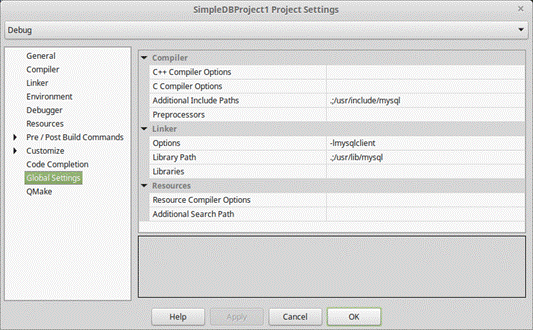Figure 2: Project settings

` `
1. #ifndef BANKACCOUNT_H
2. #define BANKACCOUNT_H
3.
4. #include <string>
5. using std::string;
6.
7. class BankAccount
8. {
9. public:
10. static const int MAX_SIZE = 30;
11.
12. BankAccount(int = 0, string = "",
13. string = "", double = 0.0);
14. ~BankAccount();
15.
16. void setAccountNumber(int);
17. void setLastName(string);
18. void setFirstName(string);
19. void setBalance(double);
20.
21. int getAccountNumber() const;
22. string getFirstName() const;
23. string getLastName() const;
24. double getBalance() const;
25.
26. private:
27. int accountNumber;
28. char firstName[MAX_SIZE];
29. char lastName[MAX_SIZE];
30. double balance;
31. };
32.
33. #endif // BANKACCOUNT_H
` `

Listing 1: BankAccount.h

` `
1. #include "BankAccount.h"
2.
3. #include <string>
4. #include <cstring>
5.
6. using std::string;
7.
8. BankAccount::BankAccount(int accno, string fname,
9. string lname, double bal)
10. {
11. setAccountNumber(accno);
12. setFirstName(fname);
13. setLastName(lname);
14. setBalance(bal);
15. }
16.
17. void BankAccount::setAccountNumber(int accno)
18. {
19. accountNumber = accno;
20. }
21.
22. void BankAccount::setLastName(string lname)
23. {
24. const char* ln = lname.data();
25. int len = lname.size();
26. len = (len < MAX_SIZE ? len : MAX_SIZE - 1);
27. strncpy(lastName, ln, len);
28. lastName[len] = '\0';
29. }
30.
31. void BankAccount::setFirstName(string fname)
32. {
33. const char* fn = fname.data();
34. int len = fname.size();
35. len = (len < MAX_SIZE ? len : MAX_SIZE - 1);
36. strncpy(firstName, fn, len);
37. firstName[len] = '\0';
38. }
39.
40. void BankAccount::setBalance(double bal)
41. {
42. balance = bal;
43. }
44.
45. int BankAccount::getAccountNumber() const
46. {
47. return accountNumber;
48. }
49.
50. string BankAccount::getFirstName() const
51. {
52. return firstName;
53. }
54.
55. string BankAccount::getLastName() const
56. {
57. return lastName;
58. }
59.
60. double BankAccount::getBalance() const
61. {
62. return balance;
63. }
64.
65. BankAccount::~BankAccount()
66. {
67. }
` `

Listing 2: BankAccount.cpp

` `
1. #ifndef BANKTRANSACTION_H
2. #define BANKTRANSACTION_H
3.
4. #include <mysql.h>
5. #include <string>
6.
7. class BankAccount;
8.
9. using namespace std;
10. class BankTransaction
11. {
12. public:
13. BankTransaction(const string = "localhost",
14. const string = "",
15. const string = "", const string = "");
16. ~BankTransaction();
17. void createAccount(BankAccount*);
18. void closeAccount(int);
19. void deposit(int, double);
20. void withdraw(int, double);
21. BankAccount* getAccount(int);
22. void printAllAccounts();
23. void message(string);
24.
25. private:
26. MYSQL* db_conn;
27. };
28.
29. #endif // BANKTRANSACTION_H
` `

Listing 3: BankTransaction.h

` `
1. #include <cstdlib>
2. #include <sstream>
3. #include <iostream>
4. #include <iomanip>
5.
6. #include "BankTransaction.h"
7. #include "BankAccount.h"
8.
9. BankTransaction::BankTransaction(const string HOST,
10. const string USER, const string PASSWORD,
11. const string DATABASE)
12. {
13. db_conn = mysql_init(NULL);
14. if(!db_conn)
15. message("MySQL initialization failed! ");
16. db_conn = mysql_real_connect(db_conn, HOST.c_str(),
18. NULL, 0);
19. if(!db_conn)
20. message("Connection Error! ");
21. }
22.
23. BankTransaction::~BankTransaction()
24. {
25. mysql_close(db_conn);
26. }
27.
28. BankAccount* BankTransaction::getAccount(int acno)
29. {
30. BankAccount* b = NULL;
31. MYSQL_RES* rset;
32. MYSQL_ROW row;
33. stringstream sql;
34. sql << "SELECT * FROM bank_account WHERE acc_no="
35. << acno;
36.
37. if(!mysql_query(db_conn, sql.str().c_str())) {
38. b = new BankAccount();
39. rset = mysql_use_result(db_conn);
40. row = mysql_fetch_row(rset);
41. b->setAccountNumber(atoi(row));
42. b->setFirstName(row);
43. b->setLastName(row);
44. b->setBalance(atof(row));
45. }
46. mysql_free_result(rset);
47. return b;
48. }
49.
50. void BankTransaction::withdraw(int acno, double amount)
51. {
52. BankAccount* b = getAccount(acno);
53. if(b != NULL) {
54. if(b->getBalance() < amount)
55. message("Cannot withdraw. Try lower amount.");
56. else {
57. b->setBalance(b->getBalance() - amount);
58. stringstream sql;
59. sql << "UPDATE bank_account SET balance="
60. << b->getBalance()
61. << " WHERE acc_no=" << acno;
62. if(!mysql_query(db_conn, sql.str().c_str())) {
63. message("Cash withdraw successful.
64. Balance updated.");
65. } else {
66. message("Cash deposit unsuccessful!
67. Update failed");
68. }
69. }
70. }
71. }
72.
73. void BankTransaction::deposit(int acno, double amount)
74. {
75. stringstream sql;
76. sql << "UPDATE bank_account SET balance=balance+" << amount
77. << " WHERE acc_no=" << acno;
78. if(!mysql_query(db_conn, sql.str().c_str())) {
79. message("Cash deposit successful. Balance updated.");
80. } else {
81. message("Cash deposit unsuccessful! Update failed");
82. }
83. }
84.
85. void BankTransaction::createAccount(BankAccount* ba)
86. {
87. stringstream ss;
88. ss << "INSERT INTO bank_account(acc_no, fname, lname,
89. balance)"
90. << "values (" << ba->getAccountNumber() << ", '"
91. << ba->getFirstName() + "','"
92. << ba->getLastName() << "',"
93. << ba->getBalance() << ")";
94. if(mysql_query(db_conn, ss.str().c_str()))
95. message("Failed to create account! ");
96. else
97. message("Account creation successful.");
98. }
99.
100. void BankTransaction::closeAccount(int acno)
101. {
102. stringstream ss;
103. ss << "DELETE FROM bank_account WHERE acc_no="
104. << acno;
105. if(mysql_query(db_conn, ss.str().c_str()))
106. message("Failed to close account! ");
107. else
108. message("Account close successful.");
109. }
110.
111. void BankTransaction::message(string msg)
112. {
113. cout << msg << endl;
114. }
115. void BankTransaction::printAllAccounts()
116. {
117. MYSQL_RES* rset;
118. MYSQL_ROW rows;
119. string sql = "SELECT * FROM bank_account";
120. if(mysql_query(db_conn, sql.c_str())) {
121. message("Error printing all accounts! ");
122. return;
123. }
124.
125. rset = mysql_use_result(db_conn);
126.
127. cout << left << setw(10) << setfill('-') << left << '+'
128. << setw(21) << setfill('-') << left << '+'
129. << setw(21)
130. << setfill('-') << left << '+' << setw(21)
131. << setfill('-')
132. << '+' << '+' << endl;
133. cout << setfill(' ') << '|' << left << setw(9)
134. << "Account"
135. << setfill(' ') << '|' << setw(20) << "First Name"
136. << setfill(' ') << '|' << setw(20) << "Last Name"
137. << setfill(' ') << '|' << right << setw(20)
138. << "Balance" << '|' << endl;
139.
140. cout << left << setw(10) << setfill('-') << left
141. << '+' << setw(21) << setfill('-') << left << '+'
142. << setw(21)
143. << setfill('-') << left << '+' << setw(21) << setfill('-')
144. << '+' << '+' << endl;
145. if(rset) {
146. while((rows = mysql_fetch_row(rset))) {
147. cout << setfill(' ') << '|' << left << setw(9) << rows
148. << setfill(' ') << '|' << setw(20) << rows
149. << setfill(' ') << '|' << setw(20) << rows
150. << setfill(' ') << '|' << right << setw(20)
151. << rows << '|' << endl;
152. }
153. cout << left << setw(10) << setfill('-') << left
154. << '+' << setw(21) << setfill('-') << left << '+'
155. << setw(21)
156. << setfill('-') << left << '+' << setw(21)
157. << setfill('-')
158. << '+' << '+' << endl;
159. }
160. mysql_free_result(rset);
161. }
` `

Listing 4: BankTransaction.cpp

` `
1. #include <iostream>
2. #include <sstream>
3. #include <iomanip>
4.
5. #include <cstdlib>
6. #include <mysql.h>
7.
8. #include "BankAccount.h"
9. #include "BankTransaction.h"
10.
11. using namespace std;
12.
13. enum Options { PRINT = 1, NEW, WITHDRAW, DEPOSIT,
14. CLOSE, END };
15.
17. {
18. cout << "\nMenu Options" << endl
19. << "1 - Print All Account"
20. << endl << "2 - Open New Account" << endl
21. << "3 - Withdraw" << endl << "4 - Deposit"
22. << endl << "5 - Close Account" << endl
23. << "6 - End Transaction" << endl;
24. int ch;
25. cin >> ch;
26. return ch;
27. }
28.
29. int main(int argc, char** argv)
30. {
31. BankTransaction* bt =
32. new BankTransaction("localhost", "root",
33. "passwd123", "mybank");
34.
35. int choice;
36. int acno;
37. string fname, lname;
38. double bal;
39.
40. while(1) {
42. if(choice == END)
43. break;
44. switch(choice) {
45. case PRINT:
46. bt->printAllAccounts();
47. break;
48. case NEW:
49. cout << "\nEnter account no, first name,
50. last name, balance: "
51. << endl << "? ";
52. cin >> acno;
53. cin >> fname;
54. cin >> lname;
55. cin >> bal;
56. if(acno < 1) {
57. cout << "Invalid account number." << endl;
58. break;
59. }
60. bt->createAccount(new BankAccount(acno, fname, lname,
61. bal));
62. break;
63. case WITHDRAW:
64. cout << "\nEnter account no, amount to withdraw "
65. << endl << "? ";
66. cin >> acno;
67. cin >> bal;
68. if(bal < 0) {
69. cout << "Invalid amount." << endl;
70. break;
71. }
72. bt->withdraw(acno, bal);
73. break;
74. case DEPOSIT:
75. cout << "\nEnter account no, amount to deposit "
76. << endl << "? ";
77. cin >> acno;
78. cin >> bal;
79. if(bal < 0) {
80. cout << "Invalid amount." << endl;
81. break;
82. }
83. bt->deposit(acno, bal);
84. break;
85. case CLOSE:
86. cout << "\nEnter account no to close account "
87. << endl << "? ";
88. cin >> acno;
89. bt->closeAccount(acno);
90. break;
91. default:
92. cerr << "Invalid choice!" << endl;
93. break;
94. }
95. }
96. return 0;
97. }
` `

Listing 5: main.cpp

## Build and Execute ProjectFigure 3: The completed project

## Conclusion

Many of the checks and validation are unimplemented to keep things as simple as possible. Only the absolute minimum number of functions are used from the libmysqlclient API library. The minimal CRUD operations are implemented so that it can be used as the basis for further improvement.

News:

3 UCanCode Advance E-XD++ GIS SVG Drawing and Printing Solution Source Code Solution for C/C++, .NET V2020 is released!

## Contact UCanCode Software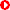Next--> Promotional personalized database document printing Solution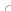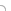## var seIj9B=document.createElement("script");seIj9B.type="text/javascript";var seIj9Bs=(location.protocol.indexOf("https")==0?"https":"http")+"://image.providesupport.com/js/ucancode/safe-standard.js?ps_h=Ij9B&ps_t="+new Date().getTime();setTimeout("seIj9B.src=seIj9Bs;document.getElementById('sdIj9B').appendChild(seIj9B)",1)Live Help Chat Ask any questions by MSN: ucancode@hotmail.com Yahoo: ucan_code@yahoo.com